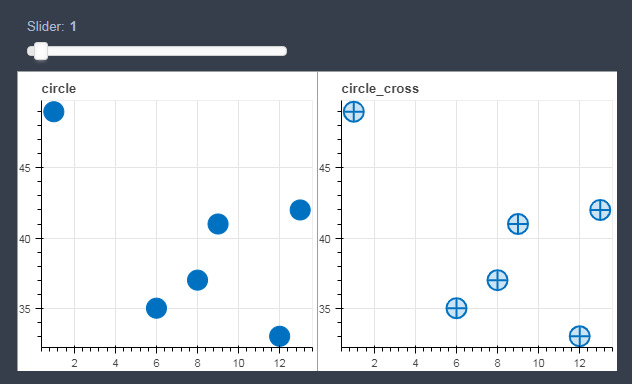# Bokeh中图形与组件的布局简介

Bokeh

Bokeh中图形与组件的布局简介

## 图形的布局

1、 column() 方法是将所有图形(plots)在一列中分布；

2、 row() 方法是将所有图形(plots)在一行中分布；

3、 gridplot() 方法，可以按需求进行行列分布。

### column

from bokeh.io import output_notebook, show
from bokeh.layouts import column, row, gridplot
from bokeh.plotting import figure
import numpy as np

output_notebook()


np.random.seed(15)

x=np.random.randint(1,20,size=6)
y=np.random.randint(20,50,size=6)

p1 = figure(title='circle',plot_width=300,plot_height=300)
p1.circle(x,y,size=20, color='#0071c1')

p2 = figure(title='circle_cross',plot_width=300,plot_height=300)
p2.circle_cross(x,y,size=20, color='#0071c1',fill_alpha=0.2, line_width=2)

p3 = figure(title='circle_x',plot_width=300,plot_height=300)
p3.circle_x(x,y,size=20, color='#0071c1',fill_alpha=0.2, line_width=2)

p4 = figure(title='cross',plot_width=300,plot_height=300)
p4.cross(x,y,size=20, color='#0071c1', line_width=2)


column_layout = column([p1, p2, p3])
show(column_layout)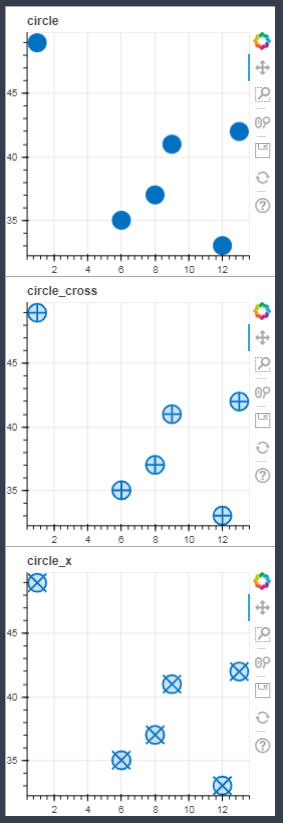### row

row_layout = row(p1,p2,p3)
show(row_layout)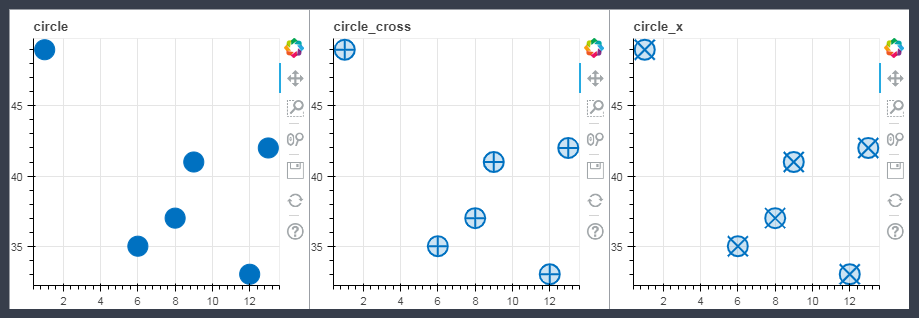### gridplot

gridplot(*args, **kwargs)

Create a grid of plots rendered on separate canvases. gridplot builds a single toolbar for all the plots in the grid. gridplot is designed to layout a set of plots. For general grid layout, use the layout() function.

Parameters:

• children (list of lists of Plot) – An array of plots to display in a grid, given as a list of lists of Plot objects. To leave a position in the grid empty, pass None for that position in the children list. OR list of Plot if called with ncols. OR an instance of GridSpec.
• sizing_mode (“fixed”, “stretch_both”, “scale_width”, “scale_height”, “scale_both”) – How will the items in the layout resize to fill the available space. Default is “fixed”. For more information on the different modes see sizing_mode description on LayoutDOM.
• toolbar_location (above, below, left, right) – Where the toolbar will be located, with respect to the grid. Default is above. If set to None, no toolbar will be attached to the grid.
• ncols (int, optional) – Specify the number of columns you would like in your grid. You must only pass an un-nested list of plots (as opposed to a list of lists of plots) when using ncols.
• plot_width (int, optional) – The width you would like all your plots to be
• plot_height (int, optional) – The height you would like all your plots to be.
• toolbar_options (dict, optional) – A dictionary of options that will be used to construct the grid’s toolbar (an instance of ToolbarBox). If none is supplied, ToolbarBox’s defaults will be used.
• merge_tools (True, False) – Combine tools from all child plots into a single toolbar.

grid1=gridplot([p1,p2],[p3,])

show(grid1)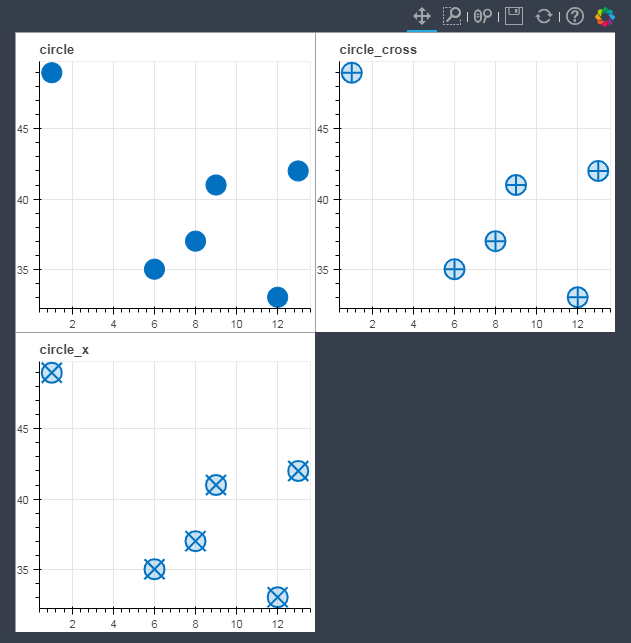grid2=gridplot([p1,p2],[None,p3])

show(grid2)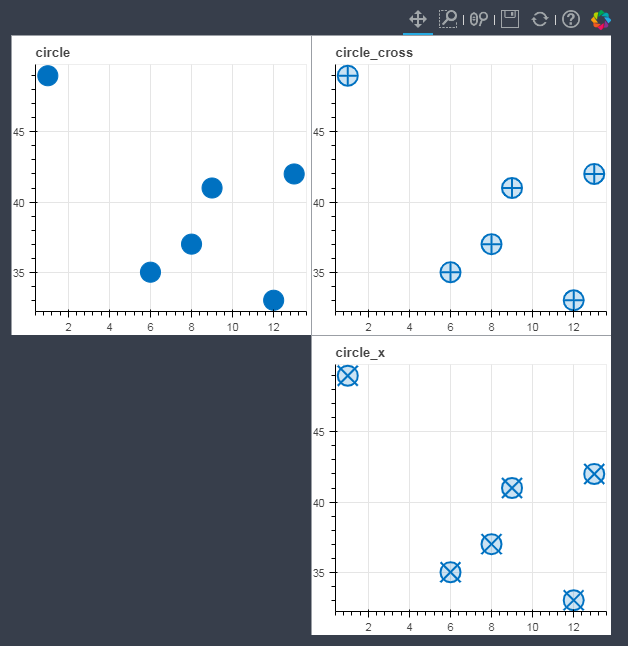P.S. 官方文档中，提到有 “ncols” 参数时，不能同时使用 “None”，但我尝试了一下，是可以同时使用 “None” 的。 有兴趣的小伙伴也可以试试。

You cannot use None with the ncols argument. It must only be a list of Plot objects at once.

grid3=gridplot([p1,p2,p4],ncols=2, plot_width=300,plot_height=300)

show(grid3)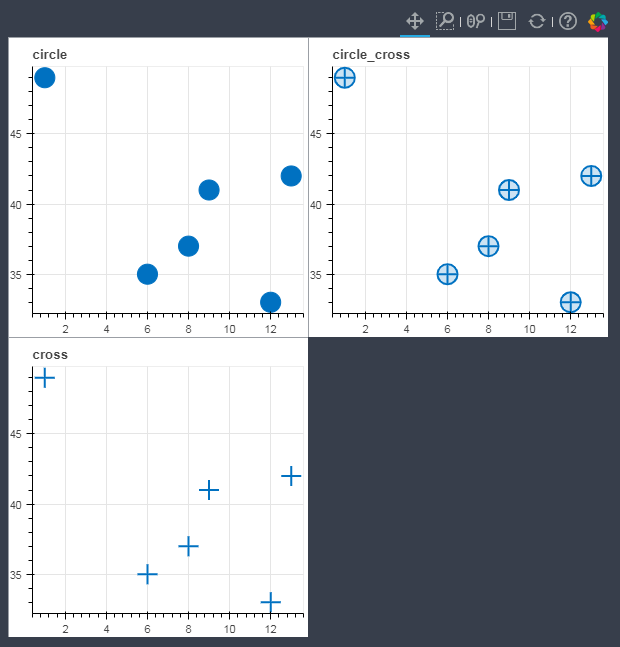grid4=gridplot([p1,p2,None,p4],ncols=2, plot_width=300,plot_height=300)

show(grid4)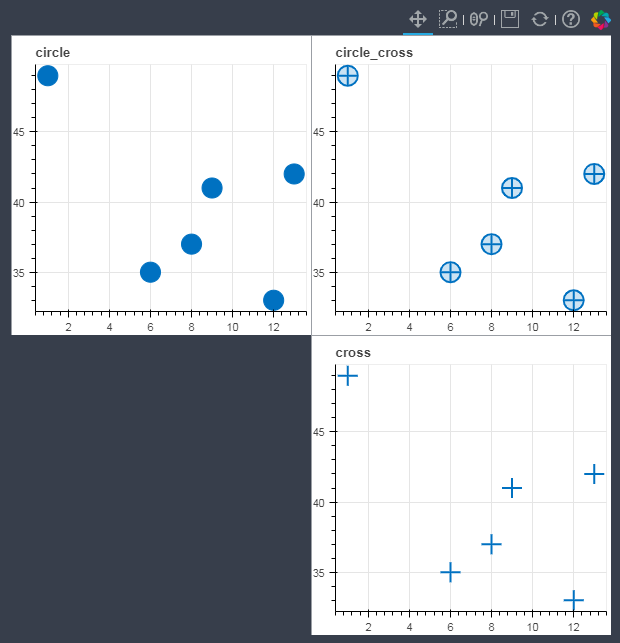## 组件的布局

bokeh 中，组件（widgets）包括 按钮（button），选项（Group），滑动块（slider）等等；组件的布局通过 widgerbox() 方法来实现

from bokeh.layouts import widgetbox
from bokeh.models.widgets import Button, RadioButtonGroup, Select, Slider
from bokeh.models.widgets import Dropdown, Toggle

# 创建一些组件
slider = Slider(start=0, end=20, value=1, step=0.5, title="Slider")
button_group = RadioButtonGroup(labels=["Option 1", "Option 2", "Option 3"], active=0)
select = Select(title="Option:", value="Lemon", options=["Lemon", "Python", "Java", "PHP"])
button_1 = Button(label="Button 1")
button_2 = Button(label="Button 2")

menu = [("Item 1", "item_1"), ("Item 2", "item_2"), None, ("Item 3", "item_3")]

# put the results in a row
show(widgetbox(button_1, slider,
button_group, select,
button_2, dropdown, width=300))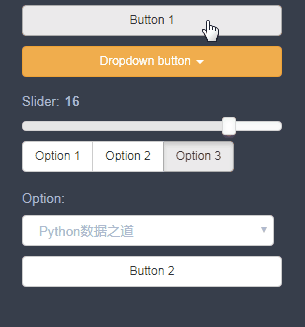## 图形和组件混合布局

from bokeh.layouts import layout

layout_01 =layout([slider],[p1,p2])

show(layout_01)